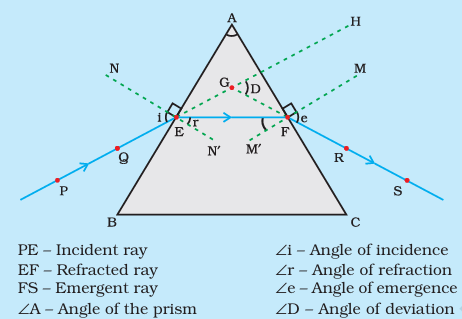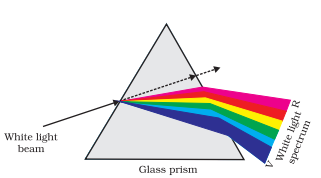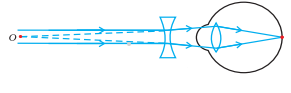# Human Eye and Colourful World Class 10 Important questions and Numericals

In this page we have Human Eye and Colourful World Class 10 Important questions and Numericals . Hope you like them and do not forget to like , social shar and comment at the end of the page.
a. One Marks Questions
b. two Marks Questions
c. Three Marks questions
d. 5 marks questions
e. Numericals

## QUESTIONS: (1 Mark each)

Question 1.
Which phenomenon is responsible for making the path of light visible?
Scattering

Question 2.
Give an example of a phenomenon where Tyndall effect can be observed.
This phenomenon is seen when a fine beam of sunlight enters a smoke-filled room through a small hole

Question 3.
What is the function of retina in the human eye?
The retina is a delicate membrane having enormous number of light-sensitive cells. The light-sensitive cells get activated upon illumination and generate electrical signals. These signals are sent to the brain via the optic nerves

Question 4.
What is iris? What is its function?
Or
Write the function of iris in the human eye.
The iris is the colored part of the eye which is behind the cornea. Iris is a dark muscular diaphragm that controls the size of the pupil and regulates and controls the amount of light entering the eye

Question 5.
What is a pupil?
The pupil is the opening in the center of the iris through which light enters the eye.

Question 6.
What kind of lens is used in the spectacles of a person suffering from myopia (near – sightedness)?
concave lens

Question 7.
To an astronaut why does the sky appear dark instead of blue?
Since there is no scattering of sunlight in space, there is no diffuse sky radiation to create the blue color that we see on Earth. The sky, therefore, appears black or dark to an astronaut.

Question 8.
What is meant by power of accommodation of the eye?
The ability of the eye lens to adjust its focal length is called accommodation

Question 9.
Which liquid fills the space between eye lens and retina?
Vitreous humour

Question 10.
What is meant by least distance of distinct vision?
The least distance of distinct vision, also known as the near point, is the minimum distance from the eye at which an object can be viewed clearly without any strain. If an object is brought closer than this distance, the eye cannot focus on it properly, and the object appears blurred.

## QUESTIONS: (2 marks each)

Question 1.
Draw a ray diagram to show the refraction of light through a glass prism. Mark on it
(a)the incident ray
(b)the emergent ray
(c)the angle of deviation.
or Draw a neat diagram to show the refraction of a light ray through a glass prism, and label on it the angle of incidence and angle of deviation.Question 2.
State the factors on which the colour of scattered light perceived by us depends.
The size of the particles in the medium relative to the wavelength of the incident light determines the type of scattering:
Particles much smaller than the wavelength scatter shorter wavelengths more, leading to a blue appearance.
Particles about the same size as the wavelength scatter all colors roughly equally, resulting in a white appearance (e.g., clouds).
Particles larger than the wavelength can cause selective scattering of certain colors, such as the blue color in colloidal solutions.

Question 3.
When ciliary muscles of a normal eye is in most contracted state. Explain with reason.
Or
If the ciliary muscles of a normal eye are in their
(a)most relaxed state.
(b)most contracted state.
Mention in brief how focal length and power of eye lens will change in both the cases. Give reason for your answer.
The ciliary muscles are ring-shaped muscles located in the eye's ciliary body. They control the shape of the eye's lens, allowing it to focus on objects at varying distances. This ability to change focus is known as accommodation.
When the ciliary muscles of a normal eye are in their most contracted state, it corresponds to the eye focusing on a nearby object.This contraction increases the curvature of the eye lens. The eye lens then becomes thicker. Consequently, the focal length of the eye lens decreases. This enables us to see nearby objects clearly.
When the ciliary muscles of a normal eye are in their most relaxed state, it corresponds to the eye focusing on a distant object.This relaxtion makes the eye lens thinner. Consequently, the focal length of the eye lens increases. This enables us to see distant objects clearly.

Question 4.
Name the part of eye where images formed in a normal human eye. State how the image position changes in myopia and hypermetropia.
Retina
In myopia,Image is formed is front of retina not at the retina
In hypermetropia,Image is formed in the back of retina not at the retina

Question 5.
What is meant by scattering of light? Use this phenomenon to explain why the clear sky appears blue or the sun appears reddish at sunrise.
Question 6.
What is meant by spectrum? How can we combine the components of white light after a prism has separated them?
Question 7.
Write approximate wavelengths for (i) blue and (ii) red light. Which colour has the higher speed in vacuum? Which colour has the higher speed in glass?

## QUESTIONS: (3Marks each)

Question 1.
Explain power of accommodation. Explain in brief the near and the far point of an eye and give their values.
The ability of the eye lens to adjust its focal length is called accommodation
The farthest point upto which the eye can see objects clearly is called the far point of the eye. It is infinity for a normal eye
The minimum distance, at which objects can be seen most distinctly without strain, is called the least distance of distinct vision. It is also called the near point of the eye.
For a young adult with normal vision, the near point is about 25 cm

Question 2.
How does the focal length of the eye lens change when we shift looking from a nearby object to a distance object?
Question 3.
Sometimes when we enter into a dark room from bright sunlight we are unable to see objects clearly. Why?
Question 4.
Define least distance of distinct vision. Give the value of least distance of distinct vision for a young adult with healthy eye.
The minimum distance, at which objects can be seen most distinctly without strain, is called the least distance of distinct vision.
For a young adult with normal vision, the near point is about 25 cm

Question 5.
What is cataract and how it can be corrected? Distinguish it from presbyopia.
Question 6.
What is a Trianglur angular prism? Explain the term dispersion of light. Illustrate with a diagram the phenomenon of dispersion through it.
It has two triangular bases and three rectangular lateral surfaces. These surfaces are inclined to each other. The angle between its two lateral faces is called the angle of the prism.
Dispersion of light is a phenomenon in optics that describes the separation of light into its constituent colors or wavelengths as it passes through a medium. This process is responsible for various beautiful natural effects, such as the formation of rainbows.
Dispersion of light occurs when a beam of white light passes through a glass prism, and is separated into its constituent colors. This separation happens because different colors of light travel at different speeds in the mediumQuestion 7.
Draw a labeled ray diagram to show the formation of rainbow in the sky giving brief explanation of the phenomena involved at each stage. List two condition necessary to observe a rainbow.
Question 8.
(a) The sky appears dark to passengers flying in space. Why?
(b) Which component of white light is least scattered by fog or smoke? Explain.

## Long Answer type (5 marks each)

Question 1.
What is atmospheric refraction? Use this phenomenon to explain the following natural events.
(a)Twinkling of stars

Question 2.
Which colour of light bends least and which one the most while passing out from the prism? Also state the reason for the same.
The red light bends the least while the violet the most.
Reason: Different colours of light bend through different angles with respect to the incident ray, as they pass through a prism

Question 3.
Explain how the components of white light can be recombined after a prism has separated them. Explain with the help of figure.

Question 4.
On the basis of this activity explain why sky appears red at sunrise or sunset.

Question 5.
What is a Tyndall effect? How is it caused? What are the conditions required to see a Tyndall effect? Give any two examples of natural phenomenon which show Tyndall effect.

Question 6.
A 14 year old student is not able to see clearly the questions written on the black board placed at a distance of 5 m from him.
(a)Name the defect of vision he is suffering from.
(b)With the help of a labelled ray diagram show how this defect can be corrected.
(c)Name the type of lens used to correct this defect.
(a) Myopia
(b)(c) Concave lens

Question 7.
What is presbyopia? Write two causes of this defect.
Question 8.
What is myopia? State the two causes of myopia. With the help of labelled ray diagrams show:
(a)the eye defect myopia.
(b)Correction of myopia using a lens
(c)Why is the normal eye unable to focus on an object placed within 10 cm from the eye?

## Numericals

Question 1.
A person suffering from an eye – defect uses lenses of power -1D to correct the defect. Name the defect is suffering from and the nature of lens to be used to correct it.
Mypopia and Concave lens

Question 2.
A person is able to see objects clearly only when these are lying at distances between 50 cm and 300 cm from his eye. Identify the kind of defect of vision he is suffering from. Give reason for your answer.
As the person is able to see objects clearly only when these are lying at distances between 50 cm and 300 cm from his eye, his near point is 50 cm and far point is 300 cm. So this person is suffering from both myopia and Hypermetropia. The far point of the person is 300 cm that’s less than infinity hence myopic. The near point of the person is 50 cm that is more than 25 cm hence the person is suffering from Hypermetropia.

Question 3.
A person needs a lens of power -6D for correcting his distant vision. For correcting his near vision, he needs a lens of power +2D.Find the focal length of the lens and its nature required for correcting distant vision and near vision.
(i) Distint vision
Power=-6D
Focal length = $\frac {1}{-6}= -.1666$ cm = $-16.66$ cm
Since it is negative, it is concave lens
(ii) Near vision
Power=2D
Focal length = $\frac {1}{2}= .50$ cm = $50$ cm
Since it is positive, it is convex lens

Question 4.
The far point of a myopic person is 150 cm in front of the eye. Calculate the focal length and the power of a lens required to enable him to see distant objects clearly.
We know from lens formula
$\frac {1}{f}= \frac {1}{v} - {1}{u}$
The image should be formed at 150cm for any distance
$\frac {1}{f}= \frac {1}{-150} - {1}{\infty}$
$f=-150 cm$
$\text{power} = \frac {1}{f}=-.67D$

Question 5.
A person is able to see objects clearly only when these are lying at distance between 60 cm and 320 cm from his eye.
1. What kind of defect of vision is he suffering from?
2. What kind of lenses will he required to increase his range of vision from 25 cm to infinity? Explain.
(i)As the person is able to see objects clearly only when these are lying at distances between 60 cm and 320 cm from his eye, his near point is 60 cm and far point is 320 cm. So this person is suffering from both myopia and Hypermetropia. The far point of the person is 300 cm that’s less than infinity hence myopic. The near point of the person is 50 cm that is more than 25 cm hence the person is suffering from Hypermetropia.
(ii)As he is sufferring from myopia and Hypermetropia,he has two options
(a) Bifocal lens: he can have a bifocal lens, where the upper portion is a concave lens to fix the myopia and the lower portion is convex lens to fix the Hypermetropia
(b) he can have two spects.One is for concave lens to fix the myopia and one is for convex lens to fix the Hypermetropia

Question 6.
A person cannot see distinctly objects kept beyond 2 m. This defect can be corrected by using a lens of power
(a) + 0.5 D
(b) – 0.5 D
(c) + 0.2 D
(d) – 0.2 D
We know from lens formula
$\frac {1}{f}= \frac {1}{v} - {1}{u}$
The image should be formed at 200cm for any distance
$\frac {1}{f}= \frac {1}{-200} - {1}{\infty}$
$f=-200 cm$
$\text{power} = \frac {1}{f}=-.5D$

Question 7.
A person needs a lens of power –4.5 D for correction of her vision.
(a) What kind of defect in vision is she suffering from?
(b) What is the focal length of the corrective lens?
(c) What is the nature of the corrective lens?
(a) Myopia
(b) Power=-4.5D
Focal length = $\frac {1}{-4.5}= -.222$ cm = $-22.22$ cm
(c)Since it is negative, it is concave lens

## Summary

This Human Eye and Colourful World Class 10 Important questions and Numericals with answers is prepared keeping in mind the latest syllabus of CBSE . This has been designed in a way to improve the academic performance of the students. If you find mistakes , please do provide the feedback on the mail.